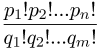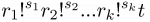## Problem F. Factorial Simplification

Peter is working on a combinatorial problem. He has carried out quite lengthy derivations and got a resulting formula that is a ratio of two products of factorials like this:$\displaystyle \frac{p_{1}!p_{2}!...p_{n}!}{q_{1}!q_{2}!...q_{m}!}$

This does not surprise Peter, since factorials appear quite often in various combinatorial formulae, because n! represents the number of transpositions of n elements - one of the basic combinatorial objects.

However, Peter might have made a mistake in his derivations. He knows that the result should be an integer number and he needs to check this first. For an integer result Peter wants to simplify this formula to get a better feeling of its actual combinatorial significance. He wants to represent the same number as a product of factorials like this.$\displaystyle r_{1}!^{s_{1}}r_{2}!^{s_{2}}...r_{k}!^{s_{k}}t$

where all ri are distinct integer numbers greater than one in the descending order (ri > ri+1 > 1), si and t are positive integers. Among all the possible representations in this form, Peter is interested in one where r1 is the largest possible number, among those in the one where s1 is the largest possible number; among those in the one where r2 is the largest possible number; among those in the one where s2 is the largest possible number; etc, until the remaining t cannot be further represented in this form. Peter does not care about the actual value of t. He wants to know what is the factorial-product part of his result.

### Input

The first line of the input contains a single integer number n and m (1 ≤ nm ≤ 1000). The second line of the input contains n integer numbers pi (1 ≤ pi ≤ 10 000) separated by spaces. The third line of the input contains m integer numbers qi (1 ≤ qi ≤ 10 000) separated by spaces.

### Output

On the first line of the output write a single integer number k. Write k = -1 if the ratio of the given factorial products is not an integer. Write k = 0 if the ratio is an integer but it cannot be represented in the desired form. Write k > 0 followed by k lines if the ratio can be represented by a factorial product as described in the problem statement. On each of the following k lines write two integers ri and si (for i = 1 ... k) separated by a space.

### Sample Input

1 2
6
4 4

### Sample Output

-1

### Sample Input

1 2
6
3 4

### Sample Output

0

### Sample Input

4 2
9 2 2 2
3 4

### Sample Output

2
7 1
2 2

Point Value: 15 (partial)
Time Limit: 1.00s
Memory Limit: 128M